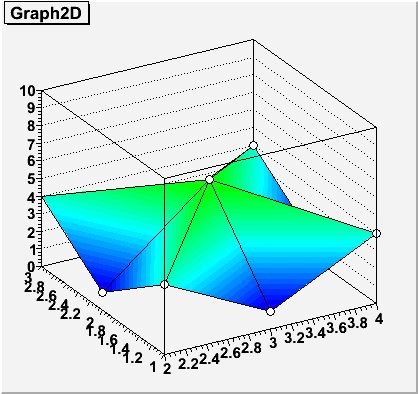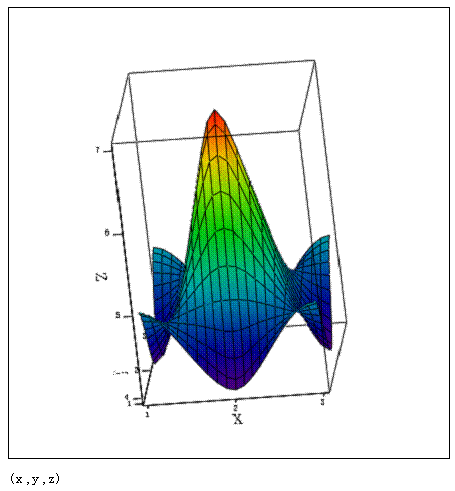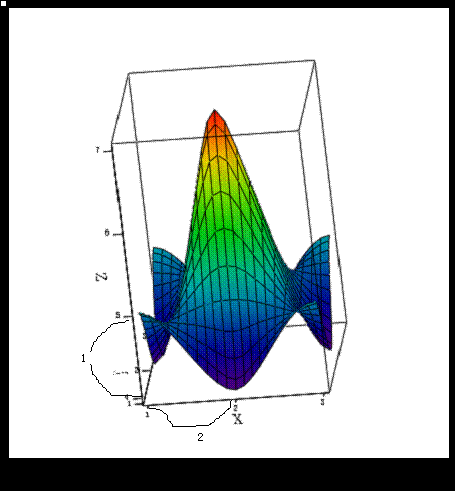# How to draw equal scales"3d color Fill Surface"

i have the data
x y z
2 3 4
2 3.5 4.5
2 4 2
2.5 3 1
2.5 3.5 3.0
2.5 4 5
3 3 2.8
3 3.5 2.7
3 4 4.6

i want to draw 3d color Fill Surface about x,y,z
but the surface can’t be activeed, why?
Thanks!

see example below:

Rene

```void gr2d() { TGraph2D *gr = new TGraph2D(); gr->SetPoint(0,2.0,3.0,4.0); gr->SetPoint(1,2.0,3.5,4.5); gr->SetPoint(2,2.0,4.0,2.0); gr->SetPoint(3,2.5,3.0,1.0); gr->SetPoint(4,2.5,3.5,3.0); gr->SetPoint(5,2.5,4.0,5.0); gr->SetPoint(6,3.0,3.0,2.8); gr->SetPoint(7,3.0,3.5,2.7); gr->SetPoint(8,3.0,4.0,4.6); gr->Draw("surf1"); } ```

void gr2d() {
TCanvas *c1 = new TCanvas(“c1”, “c1”,80,80,500,500);
//TView *view = new TView(1);
//view->SetRange(1,1,1,5,3,7);

TGraph2D *gr = new TGraph2D();
gr->SetPoint(0,2,1,4);
gr->SetPoint(1,2,2,1);
gr->SetPoint(2,2,3,4);
gr->SetPoint(3,3,1,1);
gr->SetPoint(4,3,2,6);
gr->SetPoint(5,3,3,1);
gr->SetPoint(6,4,1,4);
gr->SetPoint(7,4,2,1);
gr->SetPoint(8,4,3,4);

gStyle->SetPalette(1);

gr->Draw(“tri1 p0”);

gr->GetXaxis()->SetRangeUser(0,10);
gr->GetYaxis()->SetRangeUser(0,10);
gr->GetZaxis()->SetRangeUser(0,10);

c1->Modified();
}
i want to [color=blue]set the range of x,y,z axis from 0 to 10 . [/color]
so i write:
gr->GetXaxis()->SetRangeUser(0,10);
gr->GetYaxis()->SetRangeUser(0,10);
gr->GetZaxis()->SetRangeUser(0,10);
why
gr->GetXaxis()->SetRangeUser(0,10);
gr->GetYaxis()->SetRangeUser(0,10);
can’t run?
Thanks for you help!~!do,eg

```void gr2d() { TGraph2D *gr = new TGraph2D(); gr->SetPoint(0,2.0,3.0,4.0); gr->SetPoint(1,2.0,3.5,4.5); gr->SetPoint(2,2.0,4.0,2.0); gr->SetPoint(3,2.5,3.0,1.0); gr->SetPoint(4,2.5,3.5,3.0); gr->SetPoint(5,2.5,4.0,5.0); gr->SetPoint(6,3.0,3.0,2.8); gr->SetPoint(7,3.0,3.5,2.7); gr->SetPoint(8,3.0,4.0,4.6); TCanvas *c1 = new TCanvas("c1", "c1",80,80,500,500); TH2F *h3 = new TH2F("h3","",2,0,10,2,0,10); h3->SetMaximum(10); gStyle->SetPalette(1); gr->SetHistogram(h3); gr->Draw("tri1 p0 "); } ```
Rene

[quote=“brun”]do,eg

```void gr2d() { TGraph2D *gr = new TGraph2D(); gr->SetPoint(0,2.0,3.0,4.0); gr->SetPoint(1,2.0,3.5,4.5); gr->SetPoint(2,2.0,4.0,2.0); gr->SetPoint(3,2.5,3.0,1.0); gr->SetPoint(4,2.5,3.5,3.0); gr->SetPoint(5,2.5,4.0,5.0); gr->SetPoint(6,3.0,3.0,2.8); gr->SetPoint(7,3.0,3.5,2.7); gr->SetPoint(8,3.0,4.0,4.6); TCanvas *c1 = new TCanvas("c1", "c1",80,80,500,500); TH2F *h3 = new TH2F("h3","",2,0,10,2,0,10); h3->SetMaximum(10); gStyle->SetPalette(1); gr->SetHistogram(h3); [color=blue]gr->Draw("tri1 p0 ");[/color] } ```
Rene[/quote]

Dear Rene,thanks !
You help is important to my work!

[size=150]i want to set x,y,z axis “[color=blue]equal scales[/color]”, what should i do ?[/size]

Could you clarify what you mean by “equal scales”?
With the script above you should get equal scales.

Rene

[quote=“brun”]Could you clarify what you mean by “equal scales”?
With the script above you should get equal scales.

Rene[/quote]

in other software (mathcad) ,it names “equal scales”, perhaps i use “[color=blue][size=150]equal proportion[/size][/color]” you can know the meaning of mine.

[color=blue][size=150]what should i do ?[/size][/color]

thanks!
it is important to my work!
thank you very muchfor example , from 4 to 5 (along Z axis) "is equal to " from 1 to 2(along X axis) . The length of them are equal.

for example , from 4 to 5 (along Z axis) "is equal to " from 1 to 2(along X axis) . The length of them are equal.When will you come back to work and help me ?
Thanks!

I think you can adjust the pad size and the axis scale to get the proportions you are looking for.

TCanvas *c1 = new TCanvas(“c1”, “c1”,[size=150][color=blue]400,800[/color][/size]);
TH2F *h3 = new TH2F(“h3”,"",[size=150][color=blue]2,0,5,2,0,5[/color][/size]);
h3->SetMaximum([size=150][color=blue]10[/color][/size]);

Is it right?
Thanks!

Now when you draw a surface a lego or a TGraph2D using ROOT 3D representations in a TCanvas, the 3 axis are mapped on a cube. So even if you have very different scales on the 3 axis, the data are visible. That is what people want most of the time in HEP. But in some other domain like geography that might be not appropriate.

So, yes, as you show in your previous post you should play with the axis limits and Pad ratio to achieve what you want. In Rene’s example the 3 axis have the same lenght so if you have a square pad you will have “equal scales”. You may get the feeling that they are not the same when you rotate the plot because of the parallel projection used (here again that’s what is required most of the time).

An other possibility would be to use the openGL viewer. The perspective is much better in that case. But TGraph2D is not yet interfaced to OpenGL. That is something to be done.

Root doesn’t call this “equal scales”?